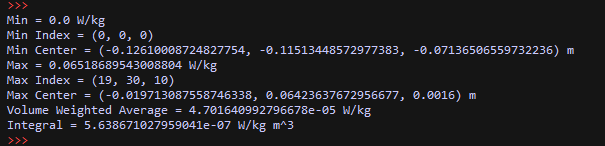# How to interpret JsonDataObject data (e.g. from Statistics evaluator)

Recently I was trying to extract the average field value using the Sim4Life Python API. Since I found interpreting the JsonDataObject a bit difficult, I will share the code here. Hope it helps.

``````# -*- coding: utf-8 -*-
# This script was auto-generated by Sim4Life version 7.1.0.8835

import numpy
import s4l_v1.analysis as analysis
import s4l_v1.document as document
import s4l_v1.model as model
import s4l_v1.units as units
from s4l_v1 import ReleaseVersion
from s4l_v1 import Unit

try:
# Define the version to use for default values
ReleaseVersion.set_active(ReleaseVersion.version7_1)

# Creating the analysis pipeline
simulation = document.AllSimulations["EM"]
simulation_extractor = simulation.Results()

em_sensor_extractor = simulation_extractor["Overall Field"]
em_sensor_extractor.FrequencySettings.ExtractedFrequency = u"All"
em_sensor_extractor.SurfaceCurrent.SurfaceResolution = 0.001, units.Meters

inputs = [em_sensor_extractor.Outputs["SAR(x,y,z,f0)"]]
statistics_evaluator = analysis.core.StatisticsEvaluator(inputs=inputs)
statistics_evaluator.Mode = u"Value"
statistics_evaluator.UpdateAttributes()
statistics_evaluator.Update()

# Get JsonDataObject output and print the values
stats = statistics_evaluator.Outputs.Data.DataSimpleDataCollection
for k in stats.Keys():
v = stats.FieldValue(k, 0)
unit = stats.FieldUnit(k)
name = stats.FieldDescription(k)
print(f"{name} = {v} {unit}")

except Exception as exc:
import traceback
traceback.print_exc()
# Reset active version to default
ReleaseVersion.reset()
raise(exc)
``````

The output is: